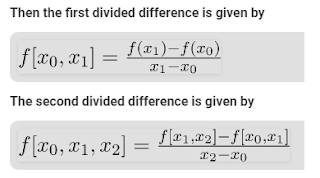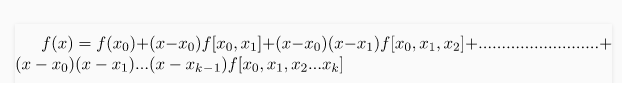# Newton Interpolation Method in Python

Interpolation is the estimation of the value of two known values   in a range of values.

Newton's fractional difference interpolation formula is an interpolation technique used when the interval difference is not equal to all values.

The (n + 1) values   of the y = f (x) function correspond to the arguments x = x0, x1, x2 are f (x0), f (x1), f (x2) ……… f (xn)… Xn, where interval differences Are not identical.### Newton Interpolation Formula### Write a Program to find value of x using Newton Interpolation Formula

value at 52

Input: x = 45, 50, 55, 60

y= f(x) = 0.7071, 0.7660, 0.8192, 0.8660

`Python Code:def u_cal(u,n):    temp = u    for i in range(1,n):        temp = temp*(u-i)    return tempdef fact(n):    f = 1    for i in range(2,n+1):        f*=i    return fn = 4x = [45,50,55,60]y = [[0 for i in range(n)] for j in range(n)]y=0.7071y=0.7660y=0.8192y=0.8660for i in range(1,n):    for j in range(n-i):        y[j][i]=y[j+1][i-1]-y[j][i-1]for i in range (n):    print(x[i],end="\t")    for j in range(n-i):        print(y[i][j],end="\t")    print("")value = 52sum = yu =(value-x/x-x)for i in range(1,n):    sum = sum+(u_cal(u,i)*y[i]/fact(i))print("\n value at",value,"is",round(sum,6))`
`Output:`
```45	0.7071	0.05890000000000006	-0.005700000000000038	-0.0007000000000000339
50	0.766	0.053200000000000025	-0.006400000000000072
55	0.8192	0.04679999999999995
60	0.866
value at 52 is 0.962846```

### Example 2:Write a program to find value of x using Newton Interpolation Formula

value at 1925
Input: x = 1891,1901,1911,1921,1931
y = f(x) = 46,66,81,93,101
```Python Codedef u_cal(u,n):     temp = u    for i in range(1,n):        temp = temp*(u-i)    return tempdef fact(n):    f = 1    for i in range(2,n+1):        f*=i    return fn = 5x = [1891,1901,1911,1921,1931]y = [[0 for i in range(n)] for j in range(n)]y=46y=66y=81y=93y=101for i in range(1,n):    for j in range(n-i):        y[j][i]=y[j+1][i-1]-y[j][i-1]for i in range (n):    print(x[i],end="\t")    for j in range(n-i):        print(y[i][j],end="\t")    print("")value=1925sum=yu =(value-x/x-x)for i in range(1,n):    sum = sum+(u_cal(u,i)*y[i]/fact(i))print("\n value at",value,"is",round(sum,6))Output:1891	46	20	-5	2	-3
1901	66	15	-3	-1
1911	81	12	-4
1921	93	8
1931	101

value at 1925 is -113859.489961```